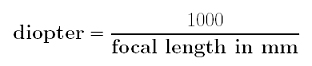Diopter and Lens Power

# Diopter and Lens PowerWhat does diopter mean? In fact, it is usually referred to as lens power. Normally, the focal length of a lens uses millimeter as a unit such as 50mm, 100mm, 8.9mm and 71.mm. The lens power or diopter is defined as follows:That is, dividing 1000 by the focal length (in mm) of a lens yields the diopter of that lens. For example, a lens of 50mm has a diopter of 1000/50 = 20, and a lens of 8.9mm has a diopter of 1000/8.9 = 112.4. Since the diopter d of a lens is usually written as +d, 50mm and 8.9mm lenses have diopters +20 and +112.4, respectively.

Conversely, if we know the diopter value of a lens, we will be able to calculate its focal length. Based on the above formula, the focal length of a lens, given its diopter, is calculated as follows:For example, if a lens has diopter +2, its focal length is 1000/2=500mm, and a lens of +4 diopter has focal length 1000/4 = 250mm.

In this way, you can easily calculate the diopter of a lens from its focal length, and the focal length of a close-up lens from its diopter value.